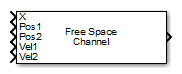# Free Space

Free space environment

## Library

Environment and Target

`phasedenvlib`## Description

The Free Space Channel block propagates the signal from one point to another in space. The block models propagation time, free space propagation loss and Doppler shift. The block assumes that the propagation speed is much greater than the target or array speed in which case the stop-and-hop model is valid.

When propagating a signal in free-space to an object and back, you have the choice of either using a single block to compute a two-way free space propagation delay or two blocks to perform one-way propagation delays in each direction. Because the free-space propagation delay is not necessarily an integer multiple of the sampling interval, it may turn out that the total round trip delay in samples when you use a two-way propagation block differs from the delay in samples when you use two one-way propagation blocks. For this reason, it is recommended that, when possible, you use a single two-way propagation block.

## Parameters

Signal Propagation speed (m/s)

Specify the propagation speed of the signal, in meters per second, as a positive scalar. You can use the function `physconst` to specify the speed of light.

Signal carrier frequency (Hz)

Specify the carrier frequency of the signal in hertz of the narrowband signal as a positive scalar.

Perform two-way propagation

Select this check box to perform round-trip propagation between the origin and destination. Otherwise the block performs one-way propagation from the origin to the destination.

Inherit sample rate

Select this check box to inherit the sample rate from upstream blocks. Otherwise, specify the sample rate using the Sample rate (Hz) parameter.

Sample rate (Hz)

Specify the signal sampling rate (in hertz) as a positive scalar. This parameter appears only when the Inherit sample rate parameter is not selected.

Maximum one-way propagation distance (m)

The maximum distance, in meters, between the origin and the destination as a positive scalar. Amplitudes of any signals that propagate beyond this distance will be set to zero.

Simulate using

Block simulation method, specified as `Interpreted Execution` or ```Code Generation```. If you want your block to use the MATLAB® interpreter, choose `Interpreted Execution`. If you want your block to run as compiled code, choose `Code Generation`. Compiled code requires time to compile but usually runs faster.

Interpreted execution is useful when you are developing and tuning a model. The block runs the underlying System object™ in MATLAB. You can change and execute your model quickly. When you are satisfied with your results, you can then run the block using ```Code Generation```. Long simulations run faster than they would in interpreted execution. You can run repeated executions without recompiling. However, if you change any block parameters, then the block automatically recompiles before execution.

When setting this parameter, you must take into account the overall model simulation mode. The table shows how the Simulate using parameter interacts with the overall simulation mode.

When the Simulink® model is in `Accelerator` mode, the block mode specified using Simulate using overrides the simulation mode.

Acceleration Modes

 Block Simulation Simulation Behavior `Normal` `Accelerator` `Rapid Accelerator` `Interpreted Execution` The block executes using the MATLAB interpreter. The block executes using the MATLAB interpreter. Creates a standalone executable from the model. `Code Generation` The block is compiled. All blocks in the model are compiled.

## Ports

### Note

The block input and output ports correspond to the input and output parameters described in the `step` method of the underlying System object. See link at the bottom of this page.

PortDescriptionSupported Data Types
`X`

Input signal.

The size of the first dimension of the input matrix can vary to simulate a changing signal length. A size change can occur, for example, in the case of a pulse waveform with variable pulse repetition frequency.

Double-precision floating point
`Pos1`

Signal origin position.

Double-precision floating point
`Pos2`

Signal destination position.

Double-precision floating point
`Vel1`

Signal origin velocity.

Double-precision floating point
`Vel2`

Signal destination velocity.

Double-precision floating point
`Out`

Output signal.

Double-precision floating point

## Algorithms

When the origin and destination are stationary relative to each other, the block output can be written as y(t) = x(t – τ)/L. The quantity τ is the delay and L is the propagation loss. The delay is computed from τ = R/c where R is the propagation distance and c is the propagation speed. The free space path loss is given by

`${L}_{fsp}=\frac{{\left(4\pi R\right)}^{2}}{{\lambda }^{2}},$`

where λ is the signal wavelength.

This formula assumes that the target is in the far-field of the transmitting element or array. In the near-field, the free-space path loss formula is not valid and can result in losses smaller than one, equivalent to a signal gain. For this reason, the loss is set to unity for range values, R ≤ λ/4π.

When there is relative motion between the origin and destination, the processing also introduces a frequency shift. This shift corresponds to the Doppler shift between the origin and destination. The frequency shift is v/λ for one-way propagation and 2v/λ for two-way propagation. The parameter v is the relative speed of the destination with respect to the origin.